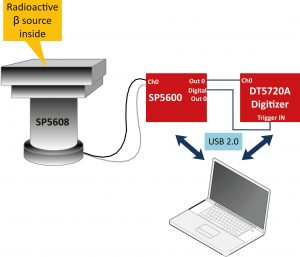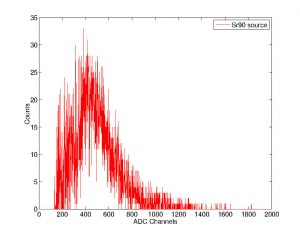Difficult Execution Time Data Analysis Radioactive SourcesNo Yes

#### Equipment

SP5600D/AN – Educational Beta Kit
Beta Radioactive Source#### Purpose of the experiment

After gamma spectrometry, the student is introduced to the measurement and interpretation of β spectra, using a plastic scintillator tile.

#### Fundamentals

There are three different beta decays:

• β decay (electron emission): n -> p+ e + νe
• β+ decay (positron emission): p -> n + e+ + νe
• Electron capture (EC): p + e -> n + νe

Where p identifies the proton, n the neutron and ν the weakly interacting neutrino. Because of the three body kinematics and the energy associated to the neutrino, the β spectrum is continuum up to a maximum energy depending on the isotope under study (and the neutrino mass).

#### Carrying out the experiment

Connect the power and the MCX cables of the SP5608 tile to one channel of the SP5600. Connect the two channel outputs to DT5720A: the analog output to the channel 0 and the digital output to “trigger IN“ of the digitizer. Use the default software values or optimize the parameters to evaluate the contribution not coming from the β source and choose the discrimination threshold in mV. After that, switch off the power supply, open the SP5608 top and place the beta source on the scintillating tile. close the support top, switch ON the power supply and acquire the beta spectrum.Experimental setup block diagram

#### Results

Measurement and interpretation of β spectra introduce the student into the field of special relativity and weak interactions of radioactive decays. Observation of the β spectrum is very important to understand the theory of β decay. Historically, experimental beta-ray spectra introduced enormous problems in the interpretation of beta decay due to the ostensible violation of the energy conservation. The introduction of neutrinos explaining the continuous β-ray spectra solved not the problem conservation of energy, momentum and lepton number. As first approach to beta spectroscopy, it is interesting to determine the maximum energy available in the decay process and to verify that the most probable energy value Eavg can be expressed as:

Eavg = 1/3 * Emax

By using several β-sources, different energy values Eavg can be estimated, each one corresponds to the total energy released in the specified β decay. An example of 90Sr spectrum is shown in the figure. For a most complete analysis on beta spectrum, other application notes are recommended.Experimental beta spectrum of 90Sr radioactive source